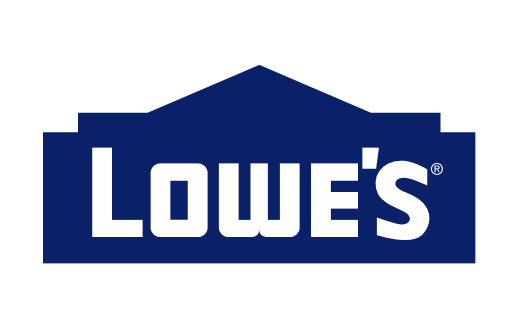Movement in arrays
Tag(s):

## Backtracking, Basic Programming, Hard, Implementation, Recursion

Problem
Editorial
Analytics

Consider an array A of size N. You start from the index 0 and your goal is to reach index $N-1$ in exactly M moves.

At any index, you can move forward or backward by a number of steps that is equal to a prime divisor of the value which exists at that index. You cannot go beyond the array while going forward or backward.

Write a program to determine whether it is possible to reach index $N-1$ in M moves.

Input format

• First line: T (number of test cases)
• First line in each test case: N
• Second line in each test case: N space-separated integers (denoting the array A)
• Third line in each test case: M

Output format

For each test case, print YES or NO depending upon the result.

Constraints

$1 \le T \le 10$
$2 \le N \le 40$
$1 \le A[i] \le 10^6$
$1 \le M \le 10^6$

SAMPLE INPUT
2
3
2 3 2
1
6
3 2 2 2 2 2
3

SAMPLE OUTPUT
YES
NO

Explanation

In testcase 1 you can directly jump from position 0 to position 2.

Time Limit: 1.0 sec(s) for each input file.
Memory Limit: 256 MB
Source Limit: 1024 KB
Marking Scheme: Marks are awarded when all the testcases pass.
Allowed Languages: Bash, C, C++, C++14, Clojure, C#, D, Erlang, F#, Go, Groovy, Haskell, Java, Java 8, JavaScript(Rhino), JavaScript(Node.js), Julia, Kotlin, Lisp, Lisp (SBCL), Lua, Objective-C, OCaml, Octave, Pascal, Perl, PHP, Python, Python 3, R(RScript), Racket, Ruby, Rust, Scala, Swift, Swift-4.1, TypeScript, Visual Basic

## CODE EDITOR

Initializing Code Editor...

## This Problem was Asked inChallenge Name

Lowe's Hiring Challenge

OTHER PROBLEMS OF THIS CHALLENGE
• Basic Programming > Implementation
• Algorithms > Graphs
• Algorithms > Graphs
• Math > Combinatorics
• Algorithms > Graphs